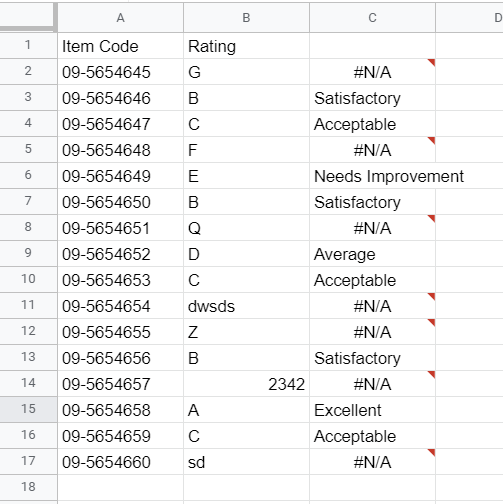# SWITCH Function: An Alternative to IF Function in Google Sheets

Learn to use this useful and valuable function which will give you greater control in altering and exchanging values when working in Google Sheets.## Review of IF Function

The IF function in Google Sheets is used for analyzing an input, applying a certain condition to it, and then selecting an output depending on whether it satisfies the condition or not. It takes the following syntax:

=IF(condition,what_happens_if_true,what_happens_if_false)

For example, if we want to apply the following conditions to the value of cell A5:

If A5>3, display “Sufficient”; else, display “Insufficient”

We will write the function as follows:

=IF(A5>3,”Sufficient”,”Insufficient”)

The IF function can also be nested. It means that you can insert the IF function inside another IF function. This is useful if you plan to apply a series of conditions into the data. To extend our example above, let’s say we add the following condition:

If A5>10, display “Excess”

Then our IF function can be written as follows:

=IF(A5>10,”Excess”,IF(A5>3,”Sufficient”,”Insufficient”))

If there are more conditions to apply, you can nest more IF functions.

If what you have is a set of “values” that serve as a switch instead of conditions, then using the IF function can be too much. For this purpose, you can use the SWITCH function.

## The SWITCH Function, Using Numbers as Input

The SWITCH function is a convenient way of applying conditional formatting when a small set of values serve as “choices” in which conditional formatting is then applied.

If a function you use outputs one of three possible values, let’s say 1, 2, and 3, and you need to convert them to their corresponding meanings AAA, BBB, and CCC, respectively, you can use the SWITCH function as follows:

=SWITCH(expression,1,”AAA”,2,”BBB”,3,”CCC”)

The expression can either be a formula or a cell reference.

For example, let’s say we have a case where, after applying data analysis, we get one of the following choices:

5 - Excellent

4 - Satisfactory

3 - Acceptable

2 - Average

1 - Needs Improvement

If, for example, we have the data on B2, we can use the switch function as follows:

=SWITCH(B2,1,"Needs Improvement",2,"Average",3,"Acceptable",4,"Satisfactory",5,"Excellent")

The output will look like this:

## The SWITCH Function, Using Letters as Input

The SWITCH function works fine if you instead have to select from a set of letters as input. When you specify the characters (or words) as input, enclose them in quotation marks.

We can modify the same example, but instead having the following classification of ratings:

A - Excellent

B - Satisfactory

C - Acceptable

D - Average

E - Needs Improvement

The SWITCH function can be used as follows:

=SWITCH(B2,"E","Needs Improvement","D","Average","C","Acceptable","B","Satisfactory","A","Excellent")

The result is now as follows:

## Adding a Catch-All Response for Unspecified Input

In data munging, you need to add functions that will indicate whether the input is already a valid input or not. We can do so using the SWITCH function.

The SWITCH function can count whether there is a one-to-one correspondence between the specified input and its corresponding output. In our previous example, note that there is a one-to-one correspondence between the specified input and its corresponding output:

=SWITCH(B2,"E","Needs Improvement","D","Average","C","Acceptable","B","Satisfactory","A","Excellent")

"E" <-> "Needs Improvement"

"D" <-> "Average"

"C" <-> "Acceptable"

"B" <-> "Satisfactory"

"A" <-> "Excellent"

If there is a space entry that has no corresponding pair, the SWITCH function can identify the last entry as the “default”. The “default” serves as the output when the actual input is not specified in the function. If a “default” is not specified, the function will display a #N/A error:SWITCH function applied to a set of letter ratings with inputs not specified in the function.

The “default” output can simply be added at the end of the SWITCH function:

=SWITCH(B2,"E","Needs Improvement","D","Average","C","Acceptable","B","Satisfactory","A","Excellent",”Invalid Input”)

The output now looks better: